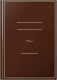Inicia la sessió
 Llibres LlibresFind an expression for the area of a triangle in terms of the coordinates of its angular points.The Principles of Analytical Geometry ... - Pàgina 72
per Henry Parr Hamilton - 1826 - 326 pàgines
Visualització completa - Sobre aquest llibre## Mathematics: From the Birth of Numbers

Jan Gullberg - 1997 - 1093 pàgines
...Cv i - ya) + *3 (yi / the vertices of the triangle may be read in any order. The formula expressing the area of a triangle in terms of the coordinates of its vertices may be applied to find the area of any polygon. Equations and Graphs of Straight Lines y =...
Previsualització limitada - Sobre aquest llibre## Field Solutions on Computers

Stanley Humphries, Jr. - 1997 - 400 pàgines
...equations will be useful for the derivation of Section 2.7. First, we shall find an expression for the area of a triangle in terms of the coordinates of its vertices. Consider a triangle lying in the xy plane with one vertex at the origin (Figure 2.10a).The...
Previsualització limitada - Sobre aquest llibre## Hungarian Problem

György Hajós, Andy Liu, G. Neukomm, János Surányi - 2001 - 142 pàgines
...the algebra can become very cumbersome. A useful result is the Coordinates-Area Formula, which gives the area of a triangle in terms of the coordinates of its vertices. If these coordinates are (xi,yi), (*2,2/2) and (X3,2/3), then the area of the triangle is...
Previsualització limitada - Sobre aquest llibre## Mathematics for Class XI, Vol. 1

Dinesh Khattar
[ El contingut d’aquesta pàgina està restringit ]
Previsualització no disponible - Sobre aquest llibre## Automated Deduction in Geometry: 5th International Workshop, ADG 2004 ...

Hoon Hong - 2006 - 211 pàgines
[ El contingut d’aquesta pàgina està restringit ]
Previsualització no disponible - Sobre aquest llibre## Proceedings of the Edinburgh Mathematical Society, Volum 11

...2/i 1 sinw d and on transformation to the other systems we readily obtain the above expressions. 4. To find the area of a triangle in terms of the co-ordinates of its angular points. (1) Independently of the corresponding expression in Cartesians. If A' denote the area, since twice...
Visualització completa - Sobre aquest llibre## Calendar

...oi+T^+ ...... is convergent if x is >1, divergent if x is = or <1. IV. CO-ORDINATE GEOMETRY. 1. Express the area of a triangle in terms of the co-ordinates of its angular points. The polar co-ordinates of three points are \3, ^J, (3, -^j, and ~ 3> 5 ) j find the rectangular co-ordinates...
Visualització completa - Sobre aquest llibre## Calendar

...of indeterminate coefficients to find the sum of the series 1.2 + 2-3 + 3-4 + ...... + n (n+1). 7. Find the area of a triangle in terms of the co-ordinates of its angular points, and explain what the resulting sign indicates. Show that the area of the triangle contained by the...
Visualització completa - Sobre aquest llibre## Curriculum for Matriculation

University of Toronto - 1931
[ El contingut d’aquesta pàgina està restringit ]
Visualització de fragments - Sobre aquest llibre## Scholarships and Prizes, Entrance Requirements ...

[ El contingut d’aquesta pàgina està restringit ]
Visualització de fragments - Sobre aquest llibre